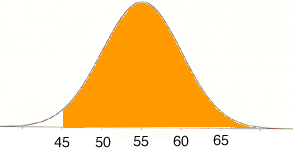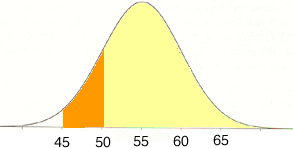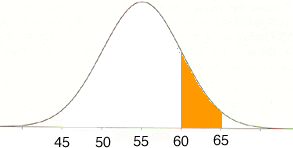SEARCH HOMEMath Central Quandaries & QueriesQuestion from cookie, a student: The attendance at football games at a certain stadium is normally distributed, with a mean of 55,000 and a standard deviation of 4,500. a) What is the probability of the attendance exceeding 60,000? b) What is the probability of the attendance being between 45,000 and 50,000? I think I got the first one right, the answer I got was 0.1335, but I'm not sure how to do the second one. Thanks, CookieYou can use the same technique to find the probability that the attendance is between 45,000 and 50,000. First find the probability that the attendance exceeds 45,000and then subtract the probability that the attendance exceeds 50,000.(the units on the horizontal axis in the diagrams are in thousands of attendees.)

If it bothers you to work to the left of the mean you can use the symmetry in the diagram to see that

Pr(45,000 < X < 50,000) = Pr(60,000 < X < 65,000)and hence find the answer to b) by subtracting from the probability that the attendance is greater than 65,000 from your answer to part a).

PennyMath Central is supported by the University of Regina and The Pacific Institute for the Mathematical Sciences.